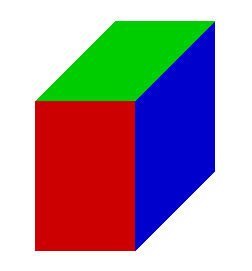# Calculate 62864

The block volume is 1440 cm3, its surface is 792 cm2, and the area of one of its walls is 92 cm2. Calculate the lengths of its sides.

a =  15.6522 cm
b =  11.2296 cm
c =  8.1927 cm

### Step-by-step explanation:Did you find an error or inaccuracy? Feel free to write us. Thank you!

Tips for related online calculators
Are you looking for help with calculating roots of a quadratic equation?